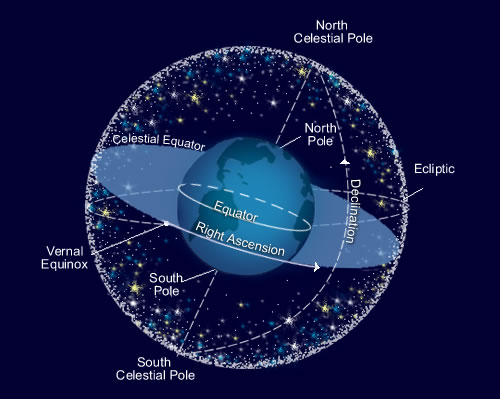## Wednesday, December 09, 2020 ...//### Celestial sphere and the anti-Wilsonian paradigm

In the mid 1990s, Andrew Strominger and Joe Polchinski would explain their $$p$$-branes and D-branes to each other during the lunches in Santa Barbara. Their brains didn't immediately notice that the ideas were equally insane in the membrane. It took some cycles of digestion to figure out that the branes were really the same thing – and a big explosion of the Second Superstring Revolution was one of the consequences.The other celestial sphere...

Can this Ansatz for a discovery be repeated? I think that Andrew Strominger (Harvard) and Nima Arkani-Hamed (IAS) have never written a paper together before – if you needed to connect them through co-author relationships, you had to go through someone like Cumrun Vafa or your humble correspondent. Today, they teamed up with two former Strominger's students and here is the first paper:

Celestial Amplitudes from UV to IR
The co-authors are Monica Pate – who has been a Harvard Junior Fellow for a year – and Ana-Maria Raclariu who is a fresh postdoc at the Perimeter Institute. And yes, the paper sort of combines Strominger's and Arkani-Hamed's industries of the recent decade. Strominger was all about the soft particles and hair that may be seen on the celestial sphere etc. (de Boer and Solodukhin studied similar things in 2003, the 2017 revival was due to Strominger, Shao, and Sabrina Speedy Gonzalez Pasterski, the pilot and aircraft constructor); Arkani-Hamed was all about the analytic structure of amplitudes, especially if expressed in twistor-like variables. The industries have been largely isolated although the overlap of the mathematical tools and hypotheses have been self-evident.

The phrase "anti-Wilsonian paradigm" in my title was taken from Section 2.1 of this new paper. They forgot to cite the original source of the anti-Wilsonian paradigm – namely the last two seconds of a parody of the Barbie Girl by Aqua. What does the phrase mean? Well, Ken Wilson became a beloved guru of particle physicists because he discovered the power of the separation of the physical phenomena according to their energy scale (or distance scale). These two ladies and two gentlemen want to become beloved for saying that you shouldn't be afraid and you should mix the physics of various scales into one giant hodgepodge.

Well, they mostly look at the four-particle scattering ($$N=4$$) in four spacetime dimensions ($$D=4$$). In the celestial sphere picture, it becomes natural to express the scattering amplitude in terms of the dimensionless $$\beta$$, the dual variable to a hyperbolic angle or pseudorapidity (the parameter multiplying the generator of the boost in the exponent expressing a Lorentz transformation). To do so, they need to consider the boost eigenstates; $$\beta$$ or $$\Delta_i$$ are symbols for the eigenvalues.

You may see that such eigenstates aren't equivalent to energy eigenstates (which are natural in Wilson's approach); they mix high energies and low energies. The transformation between energies $$\omega_i$$ and the boost angles $$\beta_i$$ is given by the Mellin transform, ${\mathcal A} (\Delta_j,z_j) = \zav{ \prod_{i=1}^n \int_0^\infty \frac{d\omega_i}{\omega_i} \omega_i^{\Delta_i} } {\bf A}(\omega_j,z_j).$ Here $$z_i$$ are the extra complex coordinates on the celestial sphere. They look at the known general short-distance (UV) and long-distance (IR) properties of the scattering amplitudes and argue that these amplitudes are meromorphic. Even negative values of $$\beta$$ are the locations of the only allowed poles; the role model for the amplitude is $$\Gamma(\beta/2)$$ although the simplest "theory" with this amplitude has some physical defects (perhaps analogous ones to bosonic string theory). Moreover, they find out that the amplitude factorizes. It may be written a simple product${\mathcal A} = {\mathcal A}_{\rm hard} {\mathcal A}_{\rm soft}$ where the soft factor is given by the low-energy spectrum (in fact, by Goldstone bosons that break some symmetries that would exist at infinity) and the hard factor has a more difficult (but still explicit, black-hole-thermodynamics-related) definition while they also find out that, due to some cancellations, ${\mathcal A}_{\rm hard} = {\mathcal A}_{\rm dressed}$ which is a simple identity that you may remember by thinking about a section of the adult servers. While the Wilsonian strategy of the separation of scales is abandoned at the beginning, this sacrifice doesn't really last indefinitely. The separation is an important principle underlying (at least the approximate) laws of physics so it has to show up. Thankfully, at the end, they translate the Wilsonian separation to an analytic property of the amplitude, anyway. At some level, I feel that they're just using "new variables" that make something easier to be seen and something is harder. Well, even if it were just that, it's probably important to get familiar with the Mellin transform and to be able to reinterpret the well-known as well as new insights in the boost-like variables. It's highly desirable to do such things because some new principles may be so original that they (or their powers) have been overlooked in the old variables.

It's an example of a paper that studies a theory of quantum gravity in the most general way, exploiting the general principles. At the same moment, the whole Ansatz is seen as a generalization of the string theory amplitude. So you will encounter the Veneziano amplitude with the "same" Gamma functions as well as its poles, the $$SL(2,\CC)$$ symmetry, and its soft high-energy behavior. Ideally, I would like to see a followup paper that would classify all possible 4D Minkowski vacua in string theory or quantum gravity (the whole landscape!) by imposing all the known constraints. Well, it's probably impossible even in principle because the four-particle amplitude doesn't necessrily know about all the constraints.